# Stable homotopy group

(diff) ← Older revision | Latest revision (diff) | Newer revision → (diff)-stable homotopy group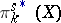, of a topological spaceThe inductive limit of the sequence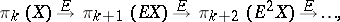(*)

where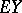is the suspension over the topological space. The suspension homomorphism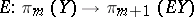relates the class of the spheroid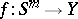to the class of the spheroid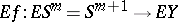, where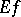is obtained by factorization from the mapping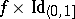. The sequence (*) stabilizes at the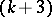-rd term (see ), so that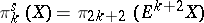.

In calculating stable homotopy groups, the Adams spectral sequence is used (see ). Up till now (1991), no stable homotopy group is known at all for any incontractible space. However, partial calculations are known for the homotopy groups of the spheres (cf. Spheres, homotopy groups of the), for an infinite-dimensional real projective space and for various other spaces.

How to Cite This Entry:
Stable homotopy group. Encyclopedia of Mathematics. URL: http://encyclopediaofmath.org/index.php?title=Stable_homotopy_group&oldid=17968
This article was adapted from an original article by D.B. Fuks (originator), which appeared in Encyclopedia of Mathematics - ISBN 1402006098. See original article